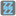• # Can I use this to solve the task ?

## Question related to missionFizz Buzz

I wrote this with my local Python2.7 and it worked well. But when I pasted it on the website, the web just kept notifying invalid syntax. What's wrong with my code? Help, plz.

print'What number do you want to put?' yes = False

while yes == False: num = input()

```if (num %3 == 0) and (num %5 == 0):
print'This is a Fizz Buzz.'
yes = True

if (num %3 != 0) and (num %5 == 0):
print'This is a Buzz.'

if (num %3 == 0) and (num %5 != 0):
print'This is a Fizz.'

if(num %3 != 0) and (num %5 != 0):
print'This is neither a Fizz nor a Buzz.'
```4